### IMO Shortlist 1995 problem G1

Kvaliteta:
Avg: 0,0
Težina:
Avg: 6,0
Let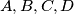$A,B,C,D$ be four distinct points on a line, in that order. The circles with diameters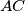$AC$ and$BD$ intersect at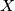$X$ and$Y$. The line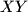$XY$ meets$BC$ at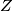$Z$. Let$P$ be a point on the line$XY$ other than$Z$. The line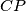$CP$ intersects the circle with diameter$AC$ at$C$ and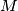$M$, and the line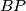$BP$ intersects the circle with diameter$BD$ at$B$ and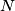$N$. Prove that the lines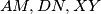$AM,DN,XY$ are concurrent.
Izvor: Međunarodna matematička olimpijada, shortlist 1995